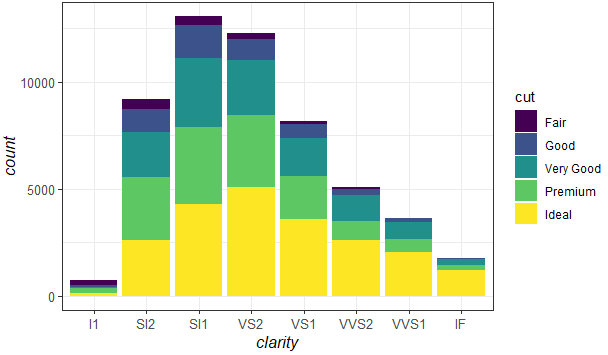2 views

I have a really simple question, which I am struggling to find the answer to. I hoped someone here might be able to help me.

An example dataframe is presented below:

a <- c(1:10)

b <- c(10:1)

df <- data.frame(a,b)

library(ggplot2)

g = ggplot(data=df) + geom_point(aes(x=a, y=b)) +

xlab("x axis")

g

I just want to learn how I change the text size of the axes titles and the axes labels.

by

To change the size of the axes title and labels in ggplot2, you can use the axis.title and axis.text in the theme function as follows:

theme(axis.text=element_text(size=16),

axis.title=element_text(size=16,face="bold"))

For example:

library("ggplot2")

ggplot(data = diamonds,aes(x = clarity)) +

geom_bar(aes(fill = cut))+

theme_bw()+

theme(axis.text=element_text(size=10),

axis.title=element_text(size=12,face="italic"))

Output: